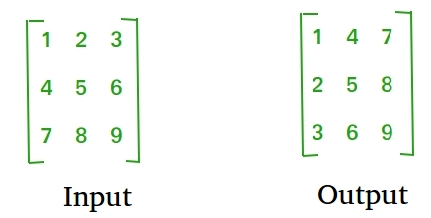GFG App
Open AppBrowser
Continue

# Java Program to Display Transpose Matrix

Transpose of a matrix is obtained by changing rows to columns and columns to rows. In other words, transpose of A[][] is obtained by changing A[i][j] to A[j][i].Approach:

• Create a 2-D Array.
• Insert the values in the array by running two nested loops. The outer ith loop will go till the no.of rows and the inner jth loop will run till the number of columns.
• For displaying the transpose of the matrix, run the same loop as explained in the above step but print the a[j[i] th element every time we are traversing inside the loop.

Example:

## Java

 `// Java Program to Display Transpose Matrix ` ` `  `import` `java.util.*; ` `public` `class` `GFG { ` `    ``public` `static` `void` `main(String args[]) ` `    ``{ ` `        ``// initialize the array of 3*3 order ` `        ``int``[][] arr = ``new` `int``[``3``][``3``]; ` ` `  `        ``System.out.println(``"enter the elements of matrix"``); ` ` `  `        ``int` `k = ``1``; ` ` `  `        ``// get the elements from user ` `        ``for` `(``int` `i = ``0``; i < ``3``; i++) { ` `            ``for` `(``int` `j = ``0``; j < ``3``; j++) { ` `                ``arr[i][j] = k++; ` `            ``} ` `        ``} ` ` `  `        ``System.out.println(``"Matrix before Transpose "``); ` ` `  `        ``// display original matrix ` `        ``for` `(``int` `i = ``0``; i < ``3``; i++) { ` `            ``for` `(``int` `j = ``0``; j < ``3``; j++) { ` `                ``System.out.print(``" "` `+ arr[i][j]); ` `            ``} ` `            ``System.out.println(); ` `        ``} ` ` `  `        ``System.out.println(``"Matrix After Transpose "``); ` ` `  `        ``// transpose and print matrix ` `        ``for` `(``int` `i = ``0``; i < ``3``; i++) { ` `            ``for` `(``int` `j = ``0``; j < ``3``; j++) { ` `                ``System.out.print(``" "` `+ arr[j][i]); ` `            ``} ` `            ``System.out.println(); ` `        ``} ` `    ``} ` `}`

Output

```enter the elements of matrix
Matrix before Transpose
1 2 3
4 5 6
7 8 9
Matrix After Transpose
1 4 7
2 5 8
3 6 9```

My Personal Notes arrow_drop_up